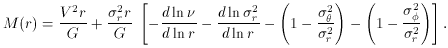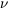3.1 Stellar Dynamical Mass Measurement

Dynamical mass measurement is conceptually simple. If random motions are small, as they are in a gas, then the mass M(r) within radius r is M(r) = V2 r / G. Here V is the rotation velocity and G is the gravitational constant. In stellar systems, some dynamical support comes from random motions, so M(r) depends also on the velocity dispersion. The measurement technique is best described in the idealized case of spherical symmetry and a velocity ellipsoid that points at the center. Then the first velocity moment of the collisionless Boltzmann equation gives(2)

Herer,, andare the radial and azimuthal components of the velocity dispersion. The densityis not the total mass density; it is the density of the luminous tracer population whose kinematics we measure. We never see, because the stars that contribute most of the light contribute almost none of the mass. Therefore we assume that(r)volume brightness. All quantities in Equation 2 are unprojected. We observe brightnesses and velocities after projection and blurring by a point-spread function (PSF). Information is lost in both processes. Several techniques have been developed to derive unprojected quantities that agree with the observations after projection and PSF convolution. From these, we derive the mass distribution M(r) and compare it to the light distribution L(r). If M/L(r) rises rapidly as r -> 0, then we have found a central dark object.

There is one tricky problem with this analysis, and it follows directly from Equation 2. Rotation and random motions contribute similarly to M(r), but the2 r / G term is multiplied by a factor that depends on the velocity anisotropy and that can be less than 1. Galaxy formation can easily produce a radial velocity dispersionr that is larger than the azimuthal componentsand. Then the third and fourth terms inside the brackets in Equation 2 are negative; they can be as small as -1 each. In fact, they can largely cancel the first two terms, because the second term cannot be larger than +1, and the first is1 in many galaxies. This explains why ad hoc anisotropic models have been so successful in explaining the kinematics of giant ellipticals without BHs. But how anisotropic are the galaxies?

Much effort has gone into finding the answer. The most powerful technique is to construct self-consistent dynamical models in which the density distribution is the linear combination=Nii of the density distributionsi of the individual orbits that are allowed by the gravitational potential. First the potential is estimated from the light distribution. Orbits of various energies and angular momenta are then calculated to construct a library of time-averaged density distributionsi. Finally, orbit occupation numbers Ni are derived so that the projected and PSF-convolved model agrees with the observed kinematics. Some authors also maximizeNi lnNi, which is analogous to an entropy. These procedures allow the stellar distribution function to be as anisotropic as it likes in order (e.g.) to try to explain the observations without a BH. In the end, such models show that real galaxies are not extremely anisotropic. That is, they do not take advantage of all the degrees of freedom that the physics would allow. However, this is not something that one could take for granted. Because the degree of anisotropy depends on galaxy luminosity, almost all BH detections in bulges and low-luminosity ellipticals (which are nearly isotropic) are based on stellar dynamics, and almost all BH detections in giant ellipticals (which are more anisotropic) are based on gas dynamics.